# Python - 变量和运算符

## 🐍常量与变量

### 🐍常量

• 程序中一直不改变的量叫常量，自从被定义了就一直不会改变。
• 但需要注意的是，Python中没有实用语法强制定义常量，一般规定用变量名全大写表明这是一个常量。然而这种方式并没有真正实现常量，对应的值仍然可以被改变。

### 🐍变量

• 变量是计算机语言中能储存计算结果或能表示值的抽象概念。
• 谈及存储就不得不提内存，内存时计算机运行时数据进行计算的地方。计算机的程序都是在内存中存储然后运行的。变量也是如此，也要存储在内存中。程序代码中需要使用这个变量运算时，这个变量才会在内存中被使用。由此，变量可以被定义为内存中分配的一块空间，在空间中保存数据，变量就是用来存储数据的。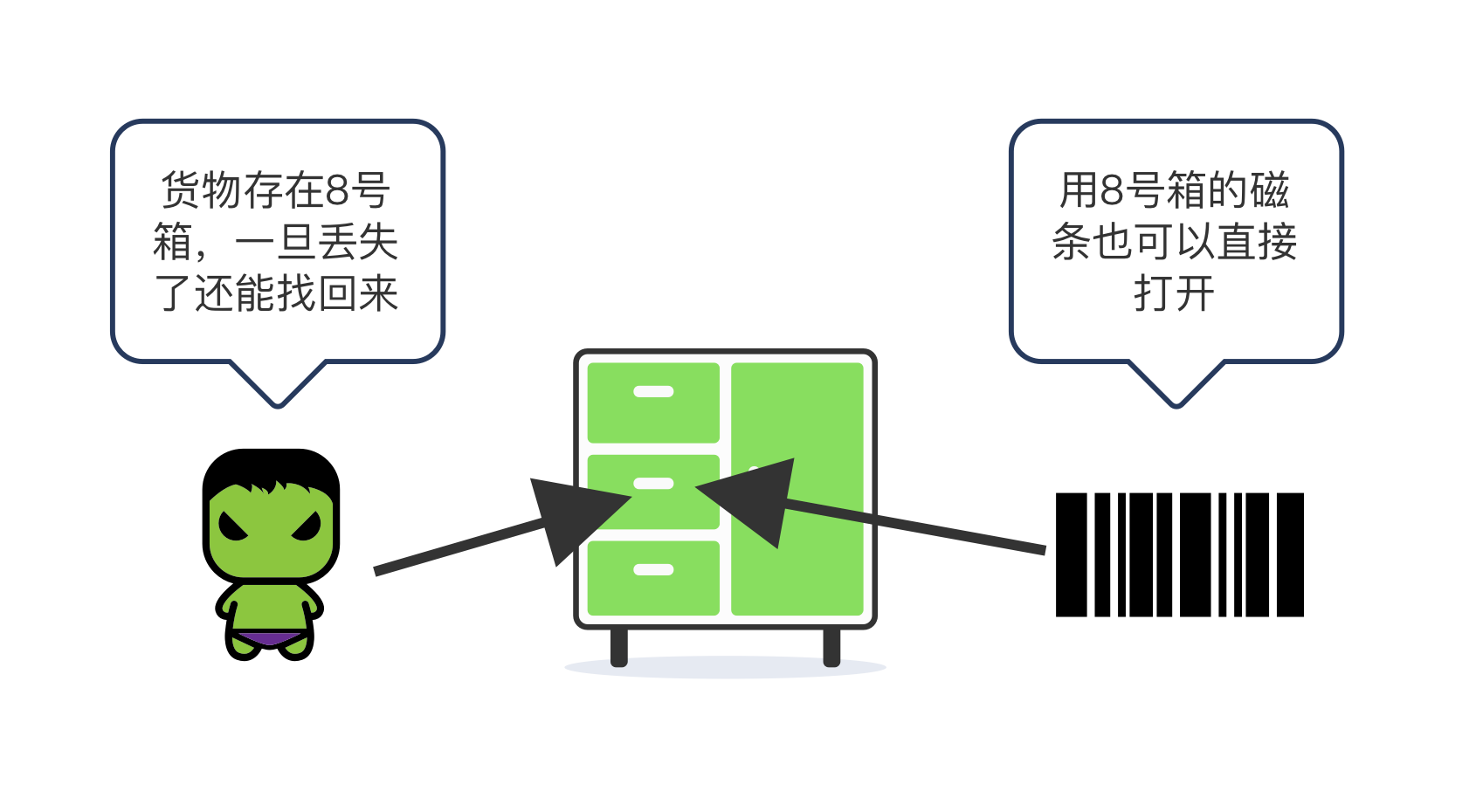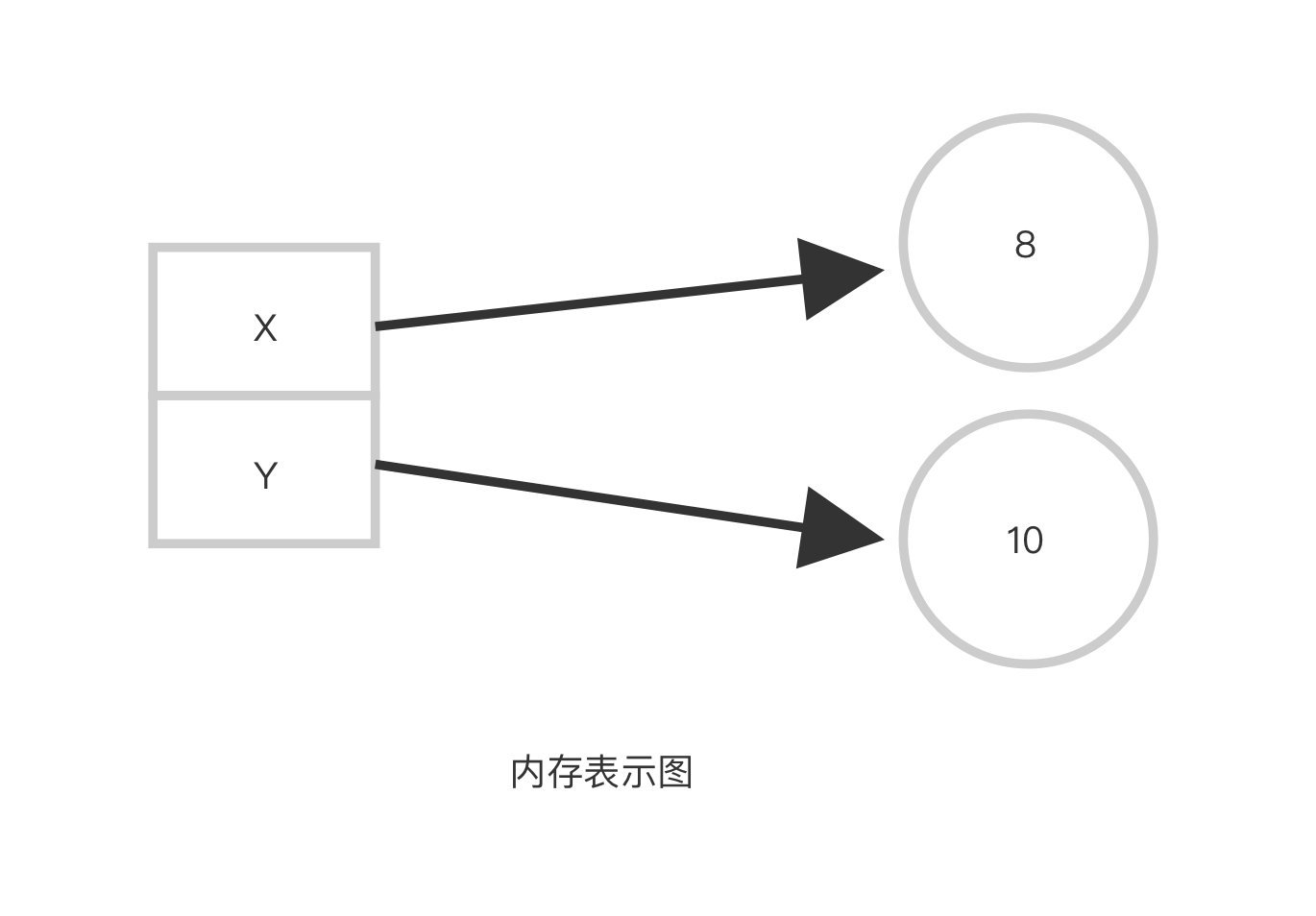x = 8
y = 8
z = 10
print("x:%d  y:%d  z:%d" %(x,y,z))
print(id(x))
print(id(y))
print(id(z))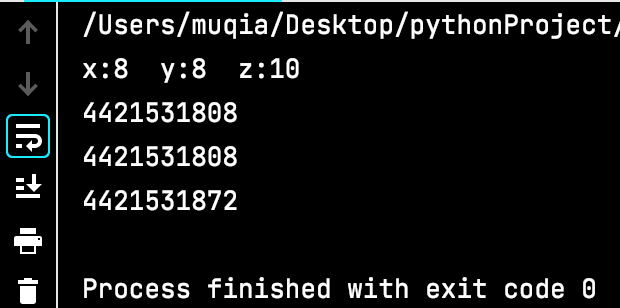## 🐍运算符

• 运算符其实就是一种功能符号，用于执行程序代码运算。
• Python支持以下类型的运算符：算术运算符、赋值运算符、逻辑运算符、关系运算符、位运算符、成员运算符、身份运算符、运算符优先级。

### 🐍算数运算符

+加，两数相加
-减，两数相减
*乘，两数相乘或返回一个被重复若干次的字符串
/除，两数相除
%取余，即返回除法的余数
//整除，即返回商的整数部分
**幂，即返回x的y次方

a = 8
b = 2
c = -3

print(a+b)
print(a-b)
print(a*b)
print(a/b)
print(a%b)
print(a//b)
print(a**b)
print("************")
print(a+c)
print(a-c)
print(a*c)
print(a/c)
print(a%c)
print(a//c)
print(a**c)
print("************")
print(c//b)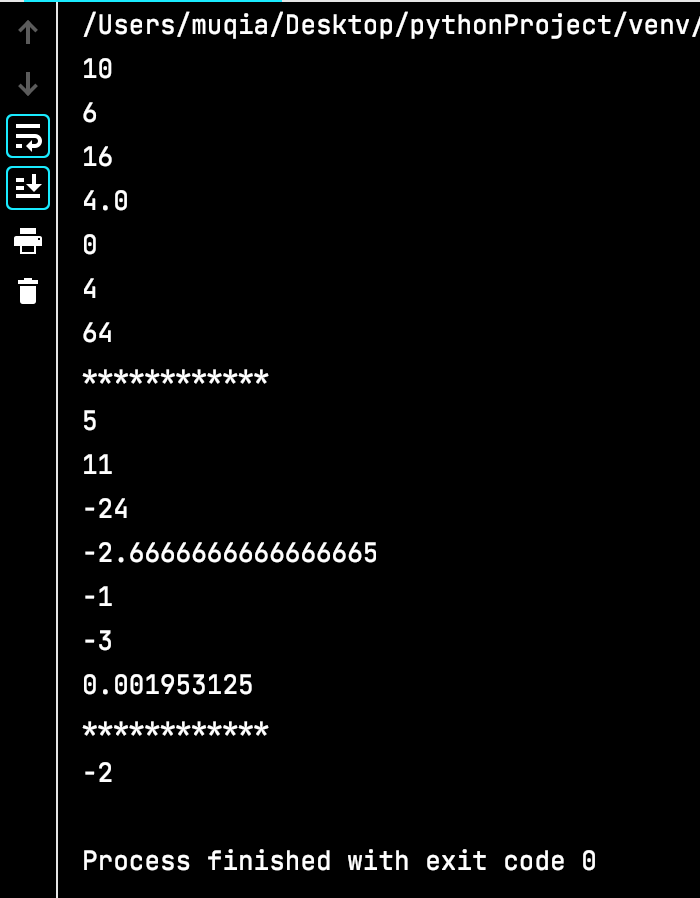### 🐍赋值运算符

=简单的赋值运算
+=加赋值
-=减赋值
*=乘赋值
/=除赋值
%=取余数赋值
//=取整除赋值
**=幂赋值

a = 0
b = 2
c = 3
d = 4

a += b  #相当于 a=a+b
print(a,b,c,d)
a -= b  #相当于 a=a-b
print(a,b,c,d)
d *= b  #相当于 d=d*b
print(a,b,c,d)
d /= b  #相当于 d=d/b
print(a,b,c,d)
d %= c  #相当于 d=d%c
print(a,b,c,d)
d //= b #相当于 d=d//b
print(a,b,c,d)
c **= b #相当于 c=c**b
print(a,b,c,d)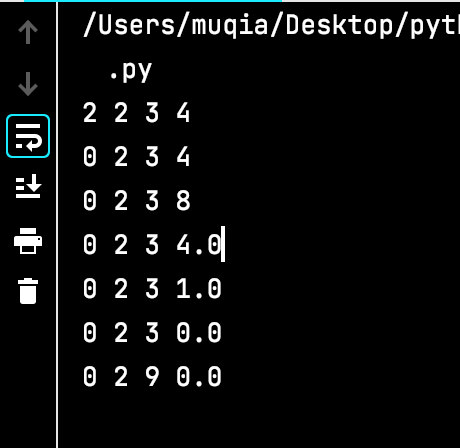### 🐍逻辑运算符

0 为 False，1为True

and布尔“与”，x为0，x and y 返回0，否则返回y的值
or布尔“与”，x or y ，x、y有一个不为0返回1，否则返回0
not布尔“非” ，如果 x 为 True，返回 False ，如果 x 为 False，返回 True

x = 1
y = 2
z = 0
print(x and y)
print(x or y)

print(x and z)
print(x or z)

print(not x)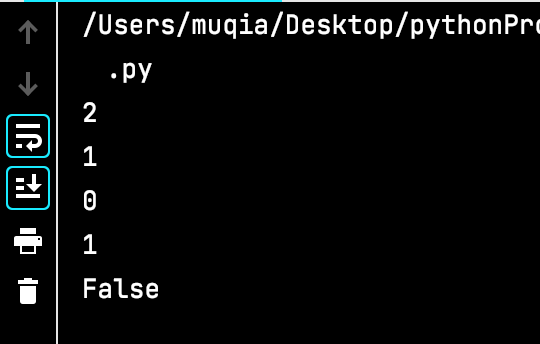### 🐍关系运算符

==等于-比较对象是否相等
！=不等于-比较对象是否不相等
>大于-返回前者是否大于后者
<小于-返回前者是否小于后者
>=大于等于-返回前者是否大于等于后者
<=小于等于-返回前者是否小于等于后者

a = 21
b = 10
c = 0

print(a==b)
print(a!=b)
print(a>b)
print(a>=b)
print(a<b)
print(a<=b)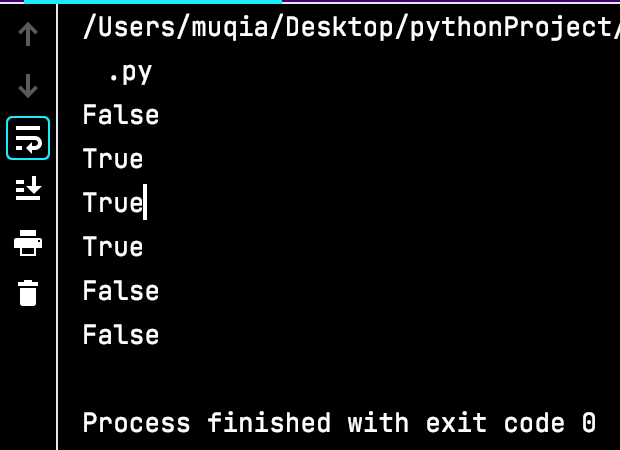### 🐍位运算符

&按位与运算符：参与运算的两个值,如果两个相应位都为1,则该位的结果为1,否则为0

^按位异或运算符：当两对应的二进位相异时，结果为1

<<左移动运算符：运算数的各二进位全部左移若干位，由"<<"右边的数指定移动的位数，高位丢弃，低位补0
>>右移动运算符：把">>“左边的运算数的各二进位全部右移若干位，”>>"右边的数指定移动的位数

a = 60  		# 60 = 0011 1100
b = 13  		# 13 = 0000 1101
c = 0

c = a & b  		# 12 = 0000 1100
print("c 的值为：", c)
c = a | b  		# 61 = 0011 1101
print("c 的值为：", c)
c = a ^ b  		# 49 = 0011 0001
print("c 的值为：", c)
c = ~a  		# -61 = 1100 0011
print("c 的值为：", c)
c = a << 2  	# 240 = 1111 0000
print("c 的值为：", c)
c = a >> 2  	# 15 = 0000 1111
print("c 的值为：", c)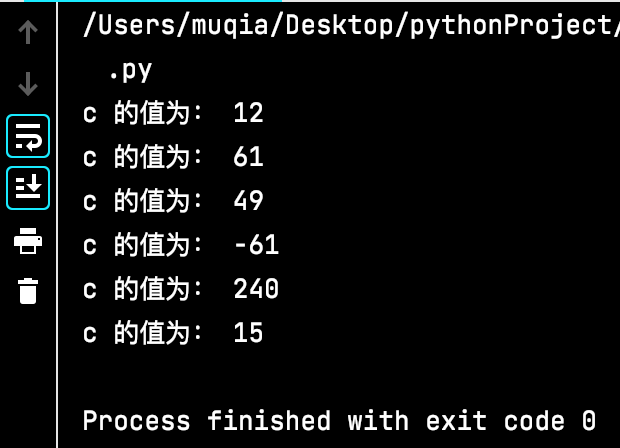### 🐍成员运算符

in如果在指定的序列中找到值返回 True，否则返回 False
not in如果在指定的序列中没有找到值返回 True，否则返回 False

a = 10
b = 2
list = [1, 2, 3, 4, 5]

print(a in list)
print(a not in list)

print(b in list)
print(b not in list)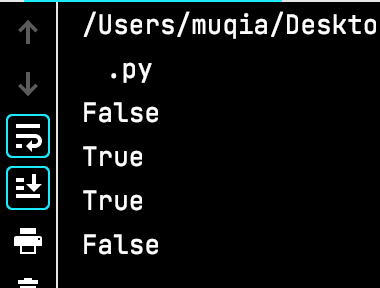### 🐍身份运算符

isis 是判断两个标识符是不是引用自一个对象，x is y, 类似 id(x) == id(y) , 如果引用的是同一个对象则返回 True，否则返回 False
is notis not 是判断两个标识符是不是引用自不同对象，x is not y ， 类似 id(a) != id(b)。如果引用的不是同一个对象则返回结果 True，否则返回 False

a = 1
b = 1
c = 2
print(a is b)
print(a is not b)
print(a is c)
print(a is not c)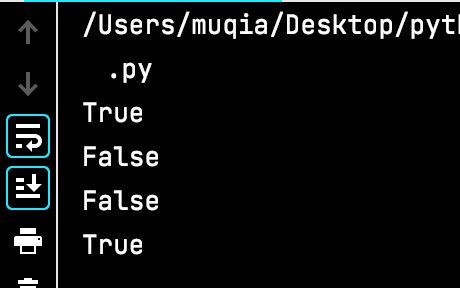### 🐍运算符优先级

**指数 (最高优先级)
~ + -按位翻转, 一元加号和减号 (最后两个的方法名为 +@ 和 -@)
* / % //乘，除，求余数和取整除
+ -加法减法
>> <<右移，左移运算符
&位 ‘AND’
^ ｜位运算符
<= < > >=比较运算符
== !=等于运算符
= %= /= //= -= += *= **=赋值运算符
is is not身份运算符
in not in成员运算符
not and or逻辑运算符，and拥有更高优先级

09-18249
07-26566
12-20541
10-18139
07-20194
02-16
08-1596
02-07232
12-19678
11-092357
12-20161
04-11599
09-1961
09-17128
09-15255

### “相关推荐”对你有帮助么？

•非常没帮助
•没帮助
•一般
•有帮助
•非常有帮助被折叠的  条评论 为什么被折叠?到【灌水乐园】发言¥1 ¥2 ¥4 ¥6 ¥10 ¥20获取中扫码支付点击重新获取扫码支付1.余额是钱包充值的虚拟货币，按照1:1的比例进行支付金额的抵扣。
2.余额无法直接购买下载，可以购买VIP、付费专栏及课程。余额充值